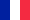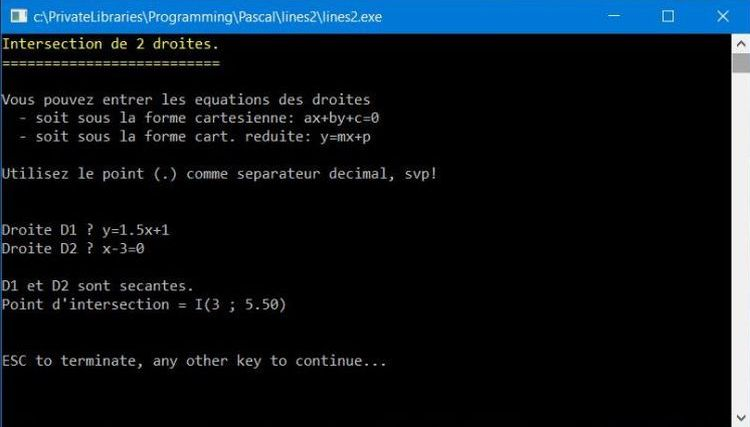# Computing: Free Pascal Programming

 Programming Home Home Contact

## Plane geometry: Parallel lines and lines intersection (lines2).Description: Geometry in the plane program, that may be used to determine if two lines are parallel (equal or distinct) or intersect (and in this case to calculate the intersection point). For user conveniance, the program allows to enter the line equation directly (instead of asking the coefficients) and this under the two common forms ax+by+c=0 and y=mx+p.

Improvements: Using the system decimal separator. Perhaps using fractions instead of decimals.

Free Pascal features: Classic pascal (Functions, procedures). Crt unit (color display, erasing given screen lines, printing at a given screen position, catching keyboard key strokes).

Screenshot: